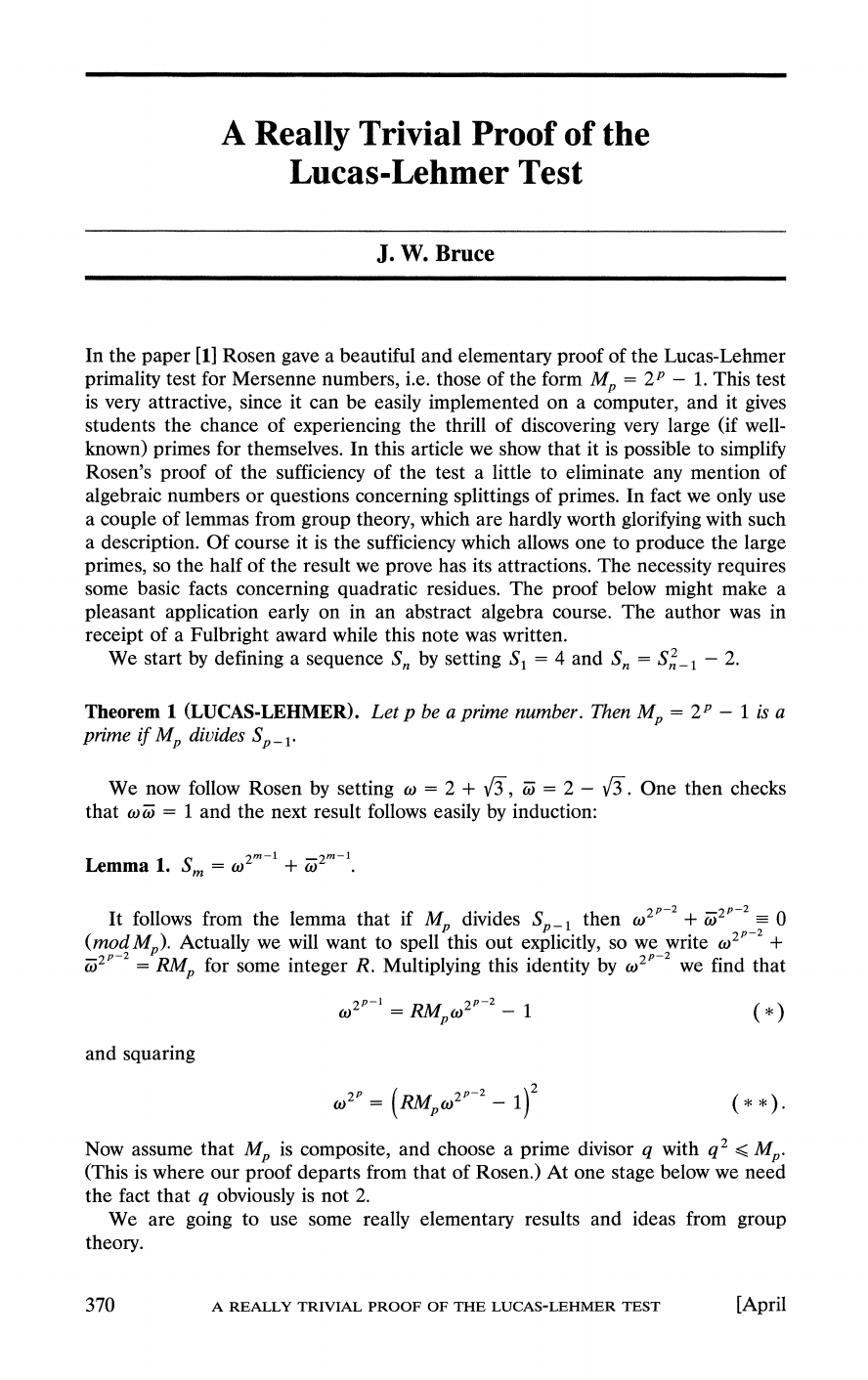The Lucas–Lehmer test (LLT) is a primality test for Mersenne number...
A basic theorem about Mersenne numbers states that if $M_p$ is prim...
In pseudocode, the test might be written as  // Determine i...
Is the theorem if and only if?
The $\textbf{overall idea of this proof}$ is to start by assuming t...
To prove that this result is equivalent to $S_m = S^2_{m-1}-2$ we j...
For $X^*$ to form a group we just need to prove the Closure propert...
The order of a group is the number of elements in that group and th...
The set of all integers which have the same remainder when divided ...
Note that $Z_q$ has $q$ elements: {$\bar 0, \bar 1, \bar 2, ..., \o... Don't forget that we chose$q$above as a prime divisor of$M_p$an... Note that$\bar w w = 1$and so$w \in X^*$Hi, I am not getting how the order of$\omega$is$2^p\$. In the...
If you want to read Rosen's original paper click [here](http://ferm...A
Really Trivial Proof of the
Lucas-Lehmer Test
J.
W. Bruce
In
the paper  Rosen gave a beautiful and elementary proof of the Lucas-Lehmer
primality test for Mersenne numbers, i.e. those of the form
M
=
2P
-
1. This test
is very attractive, since it can be easily implemented on a computer, and it gives
students the
chance
of
experiencing the
thrill
of discovering very large (if well-
known) primes
for
themselves.
In this
article we show that it is possible to simplify
Rosen's
proof
of the
sufficiency of the test a little to eliminate any mention of
algebraic numbers or questions concerning splittings of primes. In fact we only use
a
couple
of
lemmas from
group
theory,
which are hardly worth glorifying
with
such
a
description.
Of
course
it
is
the
sufficiency which allows one to produce the large
primes, so the half of the result we prove has its attractions. The necessity requires
some basic facts
residues.
The
proof below might
make a
pleasant application early
on
in
an abstract
algebra course.
The author
was
in
receipt
of a
Fulbright award while this note was written.
We start
by defining
a
sequence Sn by setting
S1
=
4
and
Sn
=
S2_1
-
2.
Theorem
1
(LUCAS-LEHMER).
Let
p
be a
prime
number. Then
Mp
=
2P
-
1
is
a
prime if
Mp
diuides
Sp_
1.
We
now
by setting
w
=
2 +
v3,
a;
=
2
-
3.
One
then checks
that wa;
=
1
and
the next result
follows
easily by
induction:
2m-1
-2m-1
Lemma
1.
Sm
=
2
+
s2m
It follows from
the lemma that
if
Mp
divides
Sp,
then 22P2
+ ai2P-2
0
(mod Mp). Actually
we
will
want
to
spell
this out
explicitly,
so we write
cv 2P2
+
=
RMp
for some integer R. Multiplying this identity by 02Pp-2
we
find that
2p-1
sM (tP-1
(2*)
and
squaring
W
2P=
(RMw 2P2
-
1) ( )
Now assume
that
Mp
is
composite,
and choose a
prime
divisor
q
with
q2
?
Mp.
(This
is where our
proof departs
from that of
Rosen.)
At
one
stage
below we need
the fact that
q obviously
is not
2.
We
are
going
to use some
really elementary
results and
ideas from
group
theory.
370
A
REALLY TRIVIAL PROOF OF THE
LUCAS-LEHMER
TEST
[April
This content downloaded from 128.240.225.120 on Fri, 20 Jun 2014 11:54:59 AM
All use subject to JSTOR Terms and Conditions